# Heat Exchanger (TL)

Heat exchanger for systems with thermal liquid and controlled flows

•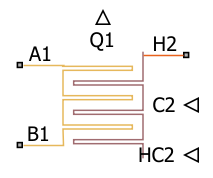Libraries:
Simscape / Fluids / Fluid Network Interfaces / Heat Exchangers

## Description

The Heat Exchanger (TL) block models the cooling and heating of fluids through conduction over a thin wall. The properties of a single-phase thermal liquid are defined on the Thermal Liquid tab. The second fluid is a controlled fluid, which is specified only by the user-defined parameters on the Controlled Fluid tab. It does not receive any properties from the domain fluid network. The heat exchange between the fluids is based on the thermal liquid sensible heat.### Block Variants

Two heat transfer models are available:

• The E-NTU Model

• The Simple Model

To set one of these models, use the Modeling option parameter.

### The `E-NTU Model` Variant

The E-NTU model, based on the Effectiveness-NTU method, is the block default variant. Steady-state heat transfer is determined based on a coefficient relating ideal to real losses in the system:

`${Q}_{\text{Act}}=ϵ{Q}_{\text{Max}},$`

where

• QAct the actual heat transfer rate.

• QMax is the ideal heat transfer rate.

• ε is the heat exchanger effectiveness, which is based on the ratio of heat capacity rates, $\frac{{C}_{\text{Min}}}{{C}_{\text{Max}}}$, and the exchanger Number of Transfer Units:

`$NTU=\frac{1}{R{C}_{\text{Min}}},$`

where R is the overall thermal resistance, which is discussed in Thermal Resistance below. CMin is the lesser heat capacity rate of the two fluids and CMax is the greater heat capacity rate of the two fluids. The heat capacity rate is calculated as $C={c}_{\text{p}}\stackrel{˙}{m}.$

Additionally, the exchanger effectiveness depends on the number of passes between the fluids and the fluid mixing conditions. For different parameterizations of ε, see E-NTU Heat Transfer. Connect an E-NTU Heat Transfer block to a Heat Exchanger (TL) block to specify the heat transfer properties in with the E-NTU method.

Flow Arrangement

Use the Flow arrangement parameter to define the flow configuration in terms of pipe orientation or effectiveness tables. When using the shell-and-tube configuration, you can select the number of passes in the exchanger. A multi-pass exchanger resembles the image below.A single-pass exchanger resembles the image below.Other flow arrangements are possible through a generic parameterization by tabulated effectiveness data. This table does not require specific heat exchanger configuration details, such as flow arrangement, mixing, and passes, for modeling the heat transfer between the fluids.

Mixing Condition

Use the Cross flow type parameter to model flows that are not restricted by baffles or walls, which homogenizes fluid temperature along the direction of flow of the second fluid and varies perpendicular to the second fluid flow. Unmixed flows vary in temperature both along and perpendicular to the flow direction of the second fluid. An example of a heat exchanger with one mixed and one unmixed fluid resembles the configuration below.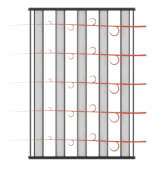A heat exchanger with two unmixed fluids resembles the configuration below.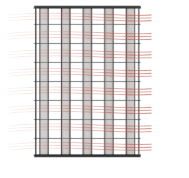In counter and parallel flow arrangements, longitudinal temperature variation in one fluid results in a longitudinal change in temperature variation in the second fluid and mixing is not taken into account.

Effectiveness Curves

Shell-and-tube exchangers with multiple passes (iv.b-e in the figure for 2, 3, and 4 passes) are the most effective type of heat exchanger. For single-pass heat exchangers, the counter-flow configuration (ii) is the most effective, and parallel flow (i) is the least.

Cross-flow exchangers are intermediate in effectiveness, with mixing condition playing a factor. They are most effective when both flows are unmixed (iii.a) and least effective when both flows are mixed (iii.b). Mixing just the flow with the smallest heat capacity rate (iii.c) lowers the effectiveness more than mixing just the flow with the largest heat capacity rate (iii.d).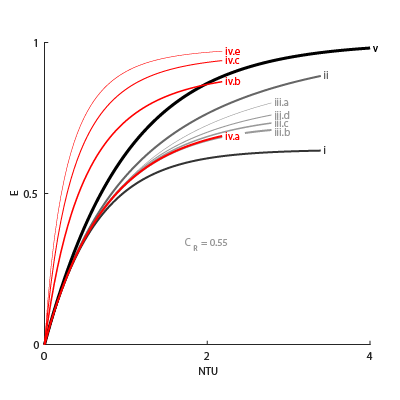Thermal Resistance

The overall thermal resistance, R, is the sum of the local resistances to heat transfer due to convection, conduction, and fouling along the heat exchanger walls:

`$R=\frac{1}{{U}_{Th}{A}_{\text{Th}}}+\frac{{F}_{Th}}{{A}_{\text{Th}}}+{R}_{\text{W}}+\frac{{F}_{\text{C}}}{{A}_{\text{C}}}+\frac{1}{{U}_{C}{A}_{\text{C}}},$`

where:

• UTh is the heat transfer coefficient between the thermal liquid and the wall.

• UC is the heat transfer coefficient between the controlled fluid and the wall, which is received as a physical signal at port HC2.

• FTh is the thermal liquid Fouling factor.

• FC is the controlled fluid Fouling factor.

• ATh is the thermal liquid Heat transfer surface area.

• AC is the controlled fluid Heat transfer surface area.

• RW is the Wall thermal resistance.The heat transfer coefficients depend on the heat exchanger configuration and fluid properties. See the E-NTU Heat Transfer reference page for more information.

Composite Structure

When the Heat Exchanger (TL) block employs the `E-NTU Model` variant, it is a composite of the Heat Exchanger Interface (TL) and E-NTU Heat Transfer blocks: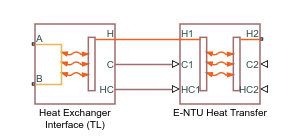### The `Simple Model` Variant

Heat transfer by the simple model is based on specific dissipation:

`$Q=\xi \left({T}_{\text{In,Th}}-{T}_{\text{In,C}}\right),$`

where:

• ξ is specific dissipation, which is a function of the mass flow rates of the thermal and controlled liquids.

• TIn,Th is the thermal liquid inlet temperature.

• TIn,C is the controlled liquid inlet temperature.

The simple model is based on linear interpolation of user-provided tabulated data and does not capture individual features of the heat exchanger.

Composite Structure

When the Heat Exchanger (TL) block employs the `Simple Model` variant, it is a composite of the Simple Heat Exchanger Interface (TL) and Specific Dissipation Heat Transfer blocks: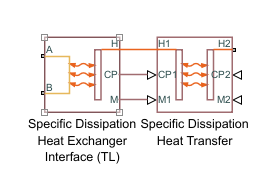## Ports

### Conserving

expand all

Opening for thermal liquid to enter and exit its side of the heat exchanger.

Opening for thermal liquid to enter and exit its side of the heat exchanger.

### Input

expand all

Instantaneous value of the heat capacity rate for the controlled flow.

#### Dependencies

This port is active only for the block variant of ```E-NTU Model```.

Instantaneous value of the heat transfer coefficient between the controlled flow and the wall.

#### Dependencies

This port is active only for the block variant of ```E-NTU Model```.

Instantaneous value of the isobaric specific heat for the controlled fluid.

#### Dependencies

This port is active only for the block variant of ```Simple Model```.

Instantaneous value of the mass flow rate of the controlled fluid.

#### Dependencies

This port is active only for the block variant of ```Simple Model```.

## Parameters

expand all

Set the heat transfer model for the block.

### Block Variant: `Simple Model`

Heat Transfer Tab

Mass flow rate of thermal liquid at each breakpoint in the lookup table for the specific heat dissipation table. The block inter- and extrapolates the breakpoints to obtain the specific heat dissipation of the heat exchanger at any mass flow rate. Interpolation is the MATLAB `linear` type and extrapolation is `nearest`.

The mass flow rates can be positive, zero, or negative, but they must increase monotonically from left to right. Their number must equal the number of columns in the Specific heat dissipation table parameter. If the table has m rows and n columns, the mass flow rate vector must be n elements long.

Mass flow rate of controlled fluid at each breakpoint in the lookup table for the specific heat dissipation table. The block inter- and extrapolates the breakpoints to obtain the specific heat dissipation of the heat exchanger at any mass flow rate. Interpolation is the MATLAB `linear` type and extrapolation is `nearest`.

The mass flow rates can be positive, zero, or negative, but they must increase monotonically from left to right. Their number must equal the number of columns in the Specific heat dissipation table parameter. If the table has m rows and n columns, the mass flow rate vector must be n elements long.

Specific heat dissipation at each breakpoint in its lookup table over the mass flow rates of thermal liquid and controlled fluid. The block inter- and extrapolates the breakpoints to obtain the effectiveness at any pair of thermal liquid and controlled fluid mass flow rates. Interpolation is the MATLAB `linear` type and extrapolation is `nearest`.

The specific heat dissipation values must be not be negative. They must align from top to bottom in order of increasing mass flow rate in the thermal liquid channel, and from left to right in order of increasing mass flow rate in the controlled fluid channel. The number of rows must equal the size of the Thermal liquid mass flow rate vector parameter, and the number of columns must equal the size of the Controlled fluid mass flow rate vector parameter.

Warning condition for specific heat dissipation in excess of minimum heat capacity rate. Heat capacity rate is the product of mass flow rate and specific heat, and its minimum value is the lowest between the flows. This minimum gives the specific dissipation for a heat exchanger with maximum effectiveness and cannot be exceeded. See the Specific Dissipation Heat Transfer block for more detail.

Pressure Loss Tab

Mass flow rate at each breakpoint in the lookup table for the pressure drop. The block inter- and extrapolates the breakpoints to obtain the pressure drop at any mass flow rate. Interpolation is the MATLAB `linear` type and extrapolation is `nearest`.

The mass flow rates can be positive, zero, or negative and they can span across laminar, transient, and turbulent zones. They must, however, increase monotonically from left to right. Their number must equal the size of the Pressure drop vector parameter, with which they are to combine to complete the tabulated breakpoints.

Pressure drop at each breakpoint in its lookup table over the mass flow rate. The block inter- and extrapolates the breakpoints to obtain the pressure drop at any mass flow rate. Interpolation is the MATLAB `linear` type and extrapolation is `nearest`.

The pressure drops can be positive, zero, or negative, and they can span across laminar, transient, and turbulent zones. They must, however, increase monotonically from left to right. Their number must equal the size of the Mass flow rate vector parameter, with which they are to combine to complete the tabulated breakpoints.

Absolute temperature established at the inlet in the gathering of the tabulated pressure drops. The reference inflow temperature and pressure determine the fluid density assumed in the tabulated data. During simulation, the ratio of reference to actual fluid densities multiplies the tabulated pressure drop to obtain the actual pressure drop.

Absolute pressure established at the inlet in the gathering of the tabulated pressure drops. The reference inflow temperature and pressure determine the fluid density assumed in the tabulated data. During simulation, the ratio of reference to actual fluid densities multiplies the tabulated pressure drop to obtain the actual pressure drop.

Mass flow rate below which its value is numerically smoothed to avoid discontinuities known to produce simulation errors at zero flow. See the Simple Heat Exchanger Interface (TL) block (on which the ```Simple Model``` variant is based) for detail on the calculations for the thermal liquid side of the exchanger.

Volume of fluid in the thermal liquid flow channel.

Flow area at the inlet and outlet of the thermal liquid flow channel. The ports are of the same size.

### Block Variant: `E-NTU Model`

Common Tab

Manner in which the flows align in the heat exchanger. The flows can run parallel to each other, counter to each other, or across each other. They can also run in a pressurized shell, one through tubes enclosed in the shell, the other around those tubes. Other flow arrangements are possible through a generic parameterization based on tabulated effectiveness data and requiring little detail about the heat exchanger.

Number of times the flow traverses the shell before exiting.

#### Dependencies

This parameter applies solely to the Flow arrangement setting of `Shell and tube`.

Mixing condition in each of the flow channels. Mixing in this context is the lateral movement of fluid as it proceeds along its flow channel toward the outlet. The flows remain separate from each other. Unmixed flows are common in channels with plates, baffles, or fins. This setting reflects in the effectiveness of the heat exchanger, with unmixed flows being most effective and mixed flows being least.

#### Dependencies

This parameter applies solely to the Flow arrangement setting of `Shell and tube`.

Number of transfer units at each breakpoint in the lookup table for the heat exchanger effectiveness number. The table is two-way, with both the number of transfer units and the thermal capacity ratio serving as independent coordinates. The block inter- and extrapolates the breakpoints to obtain the effectiveness at any number of transfer units. Interpolation is the MATLAB `linear` type and extrapolation is `nearest`.

The numbers specified must be greater than zero and increase monotonically from left to right. The size of the vector must equal the number of rows in the Effectiveness table parameter. If the table has m rows and n columns, the vector for the number of transfer units must be m elements long.

#### Dependencies

This parameter applies solely to the Flow arrangement setting of `Generic - effectiveness table`.

Thermal capacity ratio at each breakpoint in lookup table for heat exchanger effectiveness. The table is two-way, with both the number of transfer units and the heat capacity rate ratio serving as independent coordinates. The block inter- and extrapolates the breakpoints to obtain the effectiveness at any thermal capacity ratio. Interpolation is the MATLAB `linear` type and extrapolation is `nearest`.

The thermal capacity ratios must be greater than zero and increase monotonically from left to right. The size of the vector must equal the number of columns in the Nusselt number table parameter. If the table has m rows and n columns, the vector for the thermal capacity ratio must be n elements long. The thermal capacity ratio is the fraction of minimum over maximum heat capacity rates.

#### Dependencies

This parameter applies solely to the Flow arrangement setting of `Generic - effectiveness table`.

Heat exchanger effectiveness at each breakpoint in its lookup table over the number of transfer units and thermal capacity ratio. The block inter- and extrapolates the breakpoints to obtain the effectiveness at any pair of number of transfer units and thermal capacity ratio. Interpolation is the MATLAB `linear` type and extrapolation is `nearest`.

The effectiveness values must be not be negative. They must align from top to bottom in order of increasing number of transfer units and from left to right in order of increasing thermal capacity ratio. The number of rows must equal the size of the Number of heat transfer units vector parameter, and the number of columns must equal the size of the Thermal capacity ratio vector parameter.

#### Dependencies

This parameter applies solely to the Flow arrangement setting of `Generic - effectiveness table`.

Resistance of the wall to heat flow by thermal conduction, and the inverse of thermal conductance, or the product of thermal conductivity with the ratio of surface area to length. Wall resistance adds to convective and fouling resistances to determine the overall heat transfer coefficient between the flows.

Thermal liquid Tab

Cross-sectional area of the flow channel at its narrowest point. If the channel is a collection of ducts, tubes, slots, or grooves, the area is the sum of the areas in the collection—minus the occlusion due to walls, ridges, plates, or other barriers.

Total volume of fluid contained in the thermal liquid flow channel.

Effective inner diameter of the flow at its narrowest point. For channels not circular in cross section, that diameter is of an imaginary circle equal in area to the flow cross section. Its value is the ratio of the minimum free-flow area to a fourth of its gross perimeter.

If the channel is a collection of ducts, tubes, slots, or grooves, the gross perimeter is the sum of the perimeters in the collection. If the channel is a single pipe or tube and it is circular in cross section, the hydraulic diameter is the same as the true diameter.

Start of transition between laminar and turbulent zones. Above this number, inertial forces take hold and the flow grows progressively turbulent. The default value is characteristic of circular pipes and tubes with smooth surfaces.

End of transition between laminar and turbulent zones. Below this number, viscous forces take hold and the flow grows progressively laminar. The default value is characteristic of circular pipes and tubes with smooth surfaces.

Mathematical model for pressure loss by viscous friction. This setting determines which expressions to use for calculation and which block parameters to specify as input. See the Heat Exchanger Interface (TL) block for the calculations by parameterization.

Aggregate loss coefficient for all flow resistances in the flow channel—including the wall friction responsible for major loss and the local resistances, due to bends, elbows, and other geometry changes, responsible for minor loss.

The loss coefficient is an empirical dimensionless number commonly used to express the pressure loss due to viscous friction. It can be calculated from experimental data or, in some cases, obtained from product data sheets.

#### Dependencies

This parameter applies solely to the Pressure loss parameterization setting of ```Constant loss coefficient```.

Total distance the flow must travel to reach across the ports. In multi-pass shell-and-tube exchangers, the total distance is the sum over all shell passes. In tube bundles, corrugated plates, and other channels in which the flow is split into parallel branches, it is the distance covered in a single branch. The longer the flow path, the steeper the major pressure loss due to viscous friction at the wall.

#### Dependencies

This parameter applies solely to the Pressure loss parameterization setting of ```Correlations for tubes``` and ```Tabulated data - Darcy friction factor vs Reynolds number```.

Aggregate minor pressure loss expressed as a length. This length is that which all local resistances, such as elbows, tees, and unions, would add to the flow path if in their place was a simple wall extension. The larger the equivalent length, the steeper the minor pressure loss due to the local resistances.

#### Dependencies

This parameter applies solely to the Pressure loss parameterization setting of ```Correlations for tubes```.

Mean height of the surface protrusions from which wall friction arises. Higher protrusions mean a rougher wall for more friction and so a steeper pressure loss. Surface roughness features in the Haaland correlation from which the Darcy friction factor derives and on which the pressure loss calculation depends.

#### Dependencies

This parameter applies solely to the Pressure loss parameterization setting of ```Correlations for tubes```.

Pressure loss correction for flow cross section in laminar flow conditions. This parameter is commonly referred to as the shape factor. Its ratio to the Reynolds number gives the Darcy friction factor for the pressure loss calculation in the laminar zone. The default value belongs to cylindrical pipes and tubes.

The shape factor derives for certain shapes from the solution of the Navier-Stokes equations. A square duct has a shape factor of `56`, a rectangular duct with aspect ratio of 2:1 has a shape factor of `62`, and an annular tube has a shape factor of `96`, as does a slender conduit between parallel plates.

#### Dependencies

This parameter applies solely to the Pressure loss parameterization setting of ```Correlations for tubes```.

Reynolds number at each breakpoint in the lookup table for the Darcy friction factor. The block inter- and extrapolates the breakpoints to obtain the Darcy friction factor at any Reynolds number. Interpolation is the MATLAB `linear` type and extrapolation is `nearest`.

The Reynolds numbers must be greater than zero and increase monotonically from left to right. They can span across laminar, transient, and turbulent zones. Their number must equal the size of the Darcy friction factor vector parameter, with which they are to combine to complete the tabulated breakpoints.

#### Dependencies

This parameter applies solely to the Pressure loss parameterization setting of ```Tabulated data - Darcy friction factor vs. Reynolds number```.

Darcy friction factor at each breakpoint in its lookup table over the Reynolds number. The block inter- and extrapolates the breakpoints to obtain the Darcy friction factor at any Reynolds number. Interpolation is the MATLAB `linear` type and extrapolation is `nearest`.

The Darcy friction factors must not be negative and they must align from left to right in order of increasing Reynolds number. Their number must equal the size of the Reynolds number vector for Darcy friction factor parameter, with which they are to combine to complete the tabulated breakpoints.

#### Dependencies

This parameter applies solely to the Pressure loss parameterization setting of ```Tabulated data - Darcy friction factor vs. Reynolds number```.

Reynolds number at each breakpoint in the lookup table for the Euler number. The block inter- and extrapolates the breakpoints to obtain the Euler number at any Reynolds number. Interpolation is the MATLAB `linear` type and extrapolation is `nearest`.

The Reynolds numbers must be greater than zero and increase monotonically from left to right. They can span across laminar, transient, and turbulent zones. Their number must equal the size of the Euler number vector parameter, with which they are to combine to complete the tabulated breakpoints.

#### Dependencies

This parameter applies solely to the Pressure loss parameterization setting of ```Tabulated data - Euler number vs. Reynolds number```.

Euler number at each breakpoint in its lookup table over the Reynolds number. The block inter- and extrapolates the breakpoints to obtain the Euler number at any Reynolds number. Interpolation is the MATLAB `linear` type and extrapolation is `nearest`.

The Euler numbers must not be negative and they must align from left to right in order of increasing Reynolds number. Their number must equal the size of the Reynolds number vector for Euler number parameter, with which they are to combine to complete the tabulated breakpoints.

#### Dependencies

This parameter applies solely to the Pressure loss parameterization setting of ```Tabulated data - Euler number vs. Reynolds number```.

Mathematical model for heat transfer between fluid and wall. The choice of model determines which expressions to apply and which parameters to specify for heat transfer calculation. See the E-NTU Heat Transfer block for the calculations by parameterization.

Effective surface area used in heat transfer between fluid and wall. The effective surface area is the sum of primary and secondary surface areas, or those of the wall, where it is exposed to fluid, and of the fins, if any are used. Fin surface area is normally scaled by a fin efficiency factor.

Heat transfer coefficient for convection between fluid and wall. Resistance due to fouling is captured separately in the Fouling factor parameter.

#### Dependencies

This parameter applies solely to the Heat transfer parameterization setting of ```Constant heat transfer coefficient```.

Characteristic length traversed in heat transfer between fluid and wall. This length factors in the calculation of the hydraulic diameter from which the heat transfer coefficient and the Reynolds number, as defined in the tabulated heat transfer parameterizations, derives.

#### Dependencies

This parameter applies solely to the Heat transfer parameterization setting of ```Tabulated data - Colburn factor vs. Reynolds number``` or ```Tabulated data - Nusselt number vs. Reynolds number & Prandtl number```.

Constant assumed for Nusselt number in laminar flow. The Nusselt number factors in the calculation of the heat transfer coefficient between fluid and wall, on which the heat transfer rate depends. The default value belongs to cylindrical pipes and tubes.

#### Dependencies

This parameter applies solely to the Heat transfer parameterization setting of ```Correlations for tubes```.

Reynolds number at each breakpoint in the lookup table for the Colburn factor. The block inter- and extrapolates the breakpoints to obtain the Colburn factor at any Reynolds number. Interpolation is the MATLAB `linear` type and extrapolation is `nearest`.

The Reynolds numbers must be greater than zero and increase monotonically from left to right. They can span across laminar, transient, and turbulent zones. Their number must equal the size of the Colburn factor vector parameter, with which they are to combine to complete the tabulated breakpoints.

#### Dependencies

This parameter applies solely to the Heat transfer parameterization setting of ```Tabulated data - Colburn factor vs. Reynolds number```.

Colburn factor at each breakpoint in its lookup table over the Reynolds number. The block inter- and extrapolates the breakpoints to obtain the Euler number at any Reynolds number. Interpolation is the MATLAB `linear` type and extrapolation is `nearest`.

The Colburn factors must not be negative and they must align from left to right in order of increasing Reynolds number. Their number must equal the size of the Reynolds number vector for Colburn factor parameter, with which they are to combine to complete the tabulated breakpoints.

#### Dependencies

This parameter applies solely to the Heat transfer parameterization setting of ```Tabulated data - Colburn factor vs. Reynolds number```.

Reynolds number at each breakpoint in the lookup table for the Nusselt number. The table is two-way, with both Reynolds and Prandtl numbers serving as independent coordinates. The block inter- and extrapolates the breakpoints to obtain the Nusselt number at any Reynolds number. Interpolation is the MATLAB `linear` type and extrapolation is `nearest`.

The Reynolds numbers must be greater than zero and increase monotonically from left to right. They can span across laminar, transient, and turbulent zones. The size of the vector must equal the number of rows in the Nusselt number table parameter. If the table has m rows and n columns, the Reynolds number vector must be m elements long.

#### Dependencies

This parameter applies solely to the Heat transfer parameterization setting of ```Tabulated data - Nusselt number vs. Reynolds number & Prandtl number```.

Prandtl number at each breakpoint in the lookup table for the Nusselt number. The table is two-way, with both Reynolds and Prandtl numbers serving as independent coordinates. The block inter- and extrapolates the breakpoints to obtain the Nusselt number at any Prandtl number. Interpolation is the MATLAB `linear` type and extrapolation is `nearest`.

The Prandlt numbers must be greater than zero and increase monotonically from left to right. They can span across laminar, transient, and turbulent zones. The size of the vector must equal the number of columns in the Nusselt number table parameter. If the table has m rows and n columns, the Prandtl number vector must be n elements long.

#### Dependencies

This parameter applies solely to the Heat transfer parameterization setting of ```Tabulated data - Nusselt number vs. Reynolds number & Prandtl number```.

Nusselt number at each breakpoint in its lookup table over the Reynolds and Prandtl numbers. The block inter- and extrapolates the breakpoints to obtain the Nusselt number at any pair of Reynolds and Prandtl numbers. Interpolation is the MATLAB `linear` type and extrapolation is `nearest`. By determining the Nusselt number, the table feeds the calculation from which the heat transfer coefficient between fluid and wall derives.

The Nusselt numbers must be greater than zero. They must align from top to bottom in order of increasing Reynolds number and from left to right in order of increasing Prandlt numbers. The number of rows must equal the size of the Reynolds number vector for Nusselt number parameter, and the number of columns must equal the size of the Prandtl number vector for Nusselt number parameter.

#### Dependencies

This parameter applies solely to the Heat transfer parameterization setting of ```Tabulated data - Nusselt number vs. Reynolds number & Prandtl number```.

Measure of thermal resistance due to fouling deposits which over time tend to build on the exposed surfaces of the wall. The deposits, as they impose between the fluid and wall a new solid layer through which heat must traverse, add to the heat transfer path an extra thermal resistance. Fouling deposits grow slowly and the resistance due to them is accordingly assumed constant during simulation.

Lower bound for the heat transfer coefficient between fluid and wall. If calculation returns a lower heat transfer coefficient, this bound replaces the calculated value.

Controlled Fluid Tab

Aggregate heat transfer surface area on the controlled fluid side

Measure of thermal resistance due to fouling deposits which over time tend to build on the exposed surfaces of the wall. The deposits, as they impose between the controlled fluid and wall a new solid layer through which heat must traverse, add to the heat transfer path an extra thermal resistance. Fouling deposits grow slowly and the resistance due to them is accordingly assumed constant during simulation.

Lower bound for the heat transfer coefficient between the controlled fluid and wall. If calculation returns a lower heat transfer coefficient, this bound replaces the calculated value.

### Effects and Initial Conditions

Temperature in the thermal liquid channel at the start of simulation.

Pressure in the thermal liquid channel at the start of simulation.

## Version History

Introduced in R2016a